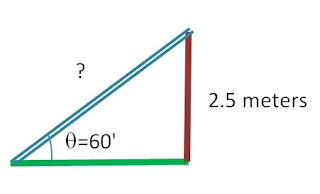### Trigonometry - Practical Real world applications and Problems in Trigonometry

#### Trigonometry :

• Trigonometry means Triangle Measurements. (Measurements of triangle)
• Considered as one of the oldest branch of mathametics as it is used since ancient days in the field of astronomy by ancient Indians and Babylonians.
• Historical records show that Babylonians used to divide a circle in 360 parts ,naming it as a degree . Maybe they had divided so, predicting 360 days for an year.
• And Indians discovered and applied sine function around 300 AD.

In modern days, trigonometry is used is real world applications in various fields like Engineering fields, Navigation purposes, Surveying applications etc.

#### Practical Application of Trigonometry :